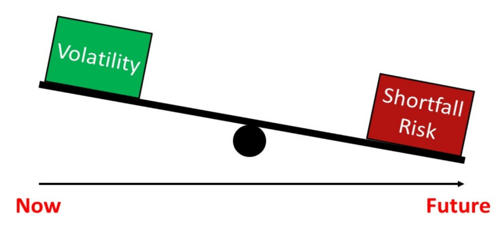Finance

# Volatility RiskVolatility risk is the risk in the value of options portfolios due to the unpredictable changes in the volatility of the underlying asset. It is the risk of a change in the price of a portfolio as a result of changes in the volatility of a risk factor. It usually applies to portfolios of derivatives instruments, where the volatility of its underlying is a major influencer of prices. It is specifically related to the breadth of the trading range between the high and low price levels at which a stock or commodity has traded.

If you trade commodities, you’ll know that volatility risk tends to be much higher than in other asset classes such as stocks. Risk is the probability that an investment will result in a permanent or long-lasting loss of value. Volatility is a statistical measure of the dispersion of returns for a given security or market index. It is merely how rapidly or significantly an investment tends to change in price over a period of time.

• Sensitivity to volatility

A measure for the sensitivity of a price of a portfolio (or asset) to changes in volatility is vega, the rate of change of the value of the portfolio with respect to the volatility of the underlying asset. Volatile assets are often considered riskier than less volatile assets because the price is expected to be less predictable. Volatility is an imperfect measure of risk, particularly in regard to the distribution of asset class returns and assumptions around correlation.

• Risk management

Volatility measures the risk of a security. It is used in option pricing formula to gauge the fluctuations in the returns of the underlying assets. This kind of risk can be managed using appropriate financial instruments whose price depends on the volatility of a given financial asset (a stock, a commodity, an interest rate, etc.). Examples are Futures contracts such as VIX for equities, or caps, floors, and swaptions for interest rates. Volatility is an important variable for calculating options prices.

Risk management is the configuration and identification of analyzing, and or acceptance during investment decision-making.  If the prices of a security fluctuate rapidly in a short time span, it is termed to have high volatility. A lower volatility means that a security’s value does not fluctuate dramatically, and tends to be more steady. If the prices of a security fluctuate slowly in a longer time span, it is termed to have low volatility. In essence, this occurs whenever an investor or portfolio manager evaluates potential losses within an investment. As volatility is a somewhat nebulous concept, it is worth considering what drives fluctuations in asset prices. Under certain investment objectives, appropriate solutions (or no solution) will occur to assess the investor’s goals and standards.